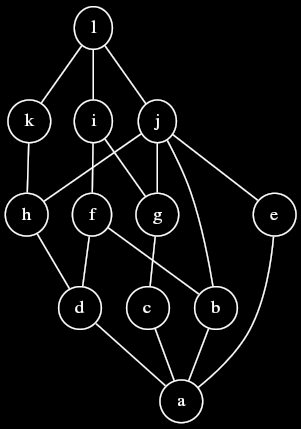# Join and meet: Exercises

Try these exercises to test your knowledge of joins and meets.

## Tangled upDetermine whether or not the following joins and meets exist in the poset depicted by the above Hasse diagram.

$$c \vee b$$

This join does not exist, because $$i$$ and $$j$$ are incomparable upper bounds of $$\{ c, b \}$$, and no smaller upper bounds of $$\{ c, b \}$$ exist.

$$g \vee e$$

This join exists. $$g \vee e = j$$.

$$j \wedge f$$

This meet does not exist, because $$d$$ and $$b$$ are incomparable lower bounds of $$\{ j, f \}$$, and no larger lower bounds of $$\{ j, f \}$$ exist.

$$\bigvee \{a,b,c,d,e,f,g,h,i,j,k,l\}$$

This join exists. It is $$l$$.

## Join fu

Let $$P$$ be a poset, $$S \subseteq P$$, and $$p \in P$$. Prove that if both $$\bigvee S$$ and $$(\bigvee S) \vee p$$ exist then $$\bigvee (S \cup \{p\})$$ exists as well, and $$(\bigvee S) \vee p = \bigvee (S \cup \{p\})$$.

For any $$X \subset P$$, let $$X^U$$ denote the set of upper bounds of $$X$$. The above proposition follows from the fact that $$\{\bigvee S, p\}^U = (S \cup p)^U$$, which is apparent from the following chain of bi-implications:

$$q \in \{\bigvee S, p\}^U \iff$$

for all $$s \in S, q \geq \bigvee S \geq s$$, and $$q \geq p \iff$$

$$q \in (S \cup \{p\})^U$$.

If two subsets of a poset have the same set of upper bounds, then either they both lack a least upper bound, or both have the same least upper bound. <div><div>

## Meet fu

Let $$P$$ be a poset, $$S \subseteq P$$, and $$p \in P$$. Prove that if both $$\bigwedge S$$ and $$(\bigwedge S) \wedge p$$ exist then $$\bigwedge (S \cup \{p\})$$ exists as well, and $$(\bigwedge S) \wedge p = \bigwedge(S \cup \{p\})$$.

Note that the proposition we are trying to prove here is the dual of the one stated in join fu. Thanks to the duality principle, this theorem therefore comes for free with our solution to join fu.

## Quite a big join

In the poset $$\langle \mathbb N, | \rangle$$ discussed in Poset: Examples, does $$\bigvee \mathbb N$$ exist? If so, what is it?